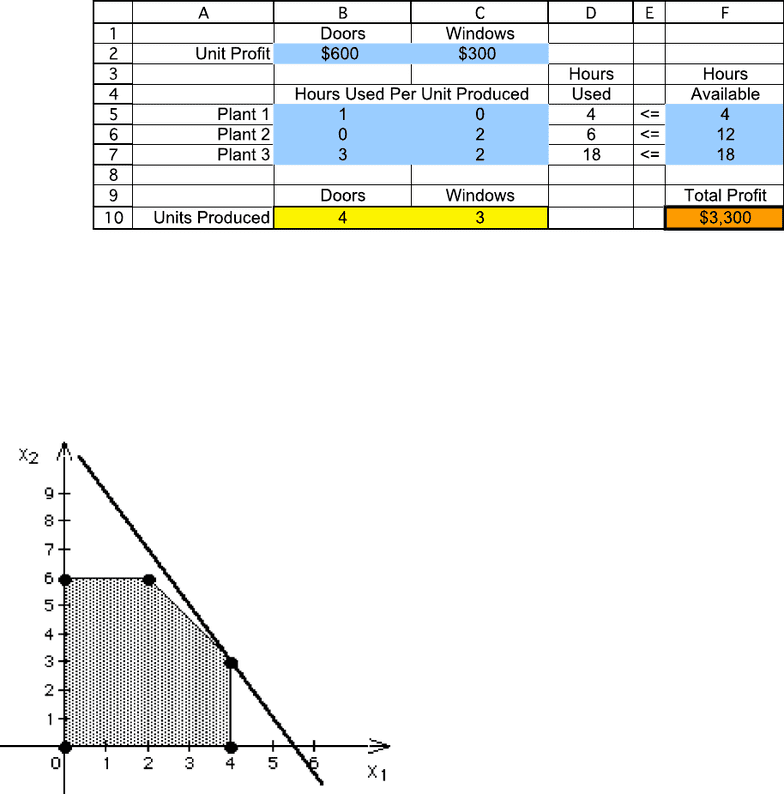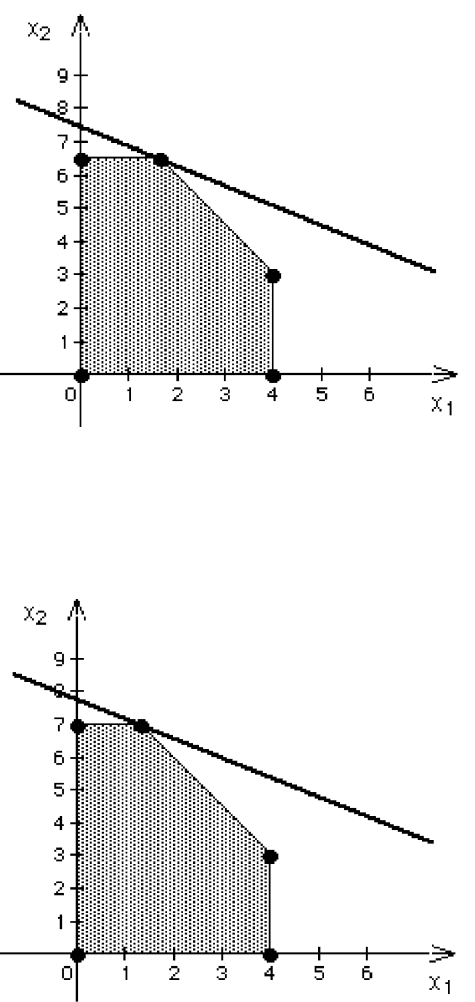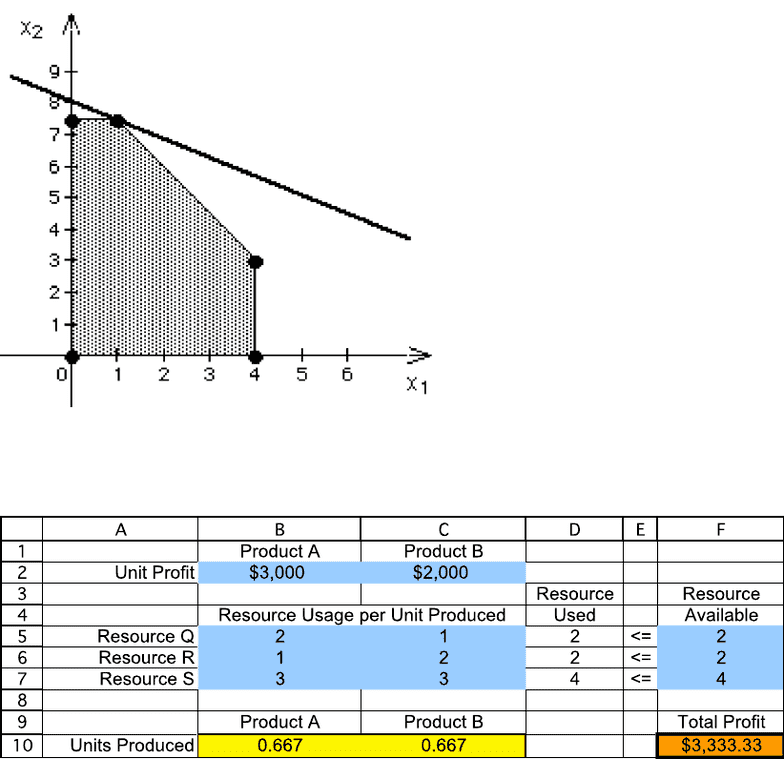Textbook Notes (280,000)
CA (170,000)
UOttawa (6,000)
Chapter 2

# ADM2302 Chapter 2: 2. Week 2 Extra Problems Solution

Department
Course Code
Professor
Rim Jaber
Chapter
2

This preview shows pages 1-3. to view the full 11 pages of the document.1
Week 2 Extra Problems Solution
CHAPTER 2
LINEAR PROGRAMMING: BASIC CONCEPTS
Problems
2.2 a)
b) Maximize P = \$600D + \$300W,
subject to D 4
2W 12
3D + 2W 18
and D ≥ 0, W ≥ 0.
c) Optimal Solution = (D, W) = (x1, x2) = (4, 3). P = \$3300.

Only pages 1-3 are available for preview. Some parts have been intentionally blurred.2
2.3 a) Optimal Solution: (D, W) = (x1, x2) = (1.67, 6.50). P = \$3750.
b) Optimal Solution: (D, W) = (x1, x2) = (1.33, 7.00). P = \$3900.

Only pages 1-3 are available for preview. Some parts have been intentionally blurred.3
c) Optimal Solution: (D, W) = (x1, x2) = (1.00, 7.50). P = \$4050.
d) Each additional hour per week would increase total profit by \$150.
2.5 a)
b) Let A = units of product A produced
B = units of product B produced
Maximize P = \$3,000A + \$2,000B,
subject to
2A + B 2
A + 2B 2
3A + 3B ≤ 4
and A ≥ 0, B ≥ 0.